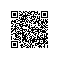# 《白话深度学习与TensorFlow》——3.5　安装TensorFlow

### 3.5　安装TensorFlow

bash Anaconda2-4.2.0-Linux-x86_64.sh


sudo reboot


conda create -n tensorflow python=2.7
source activate tensorflow


pip install tensorflow


\$ python
>>> import tensorflow as tf
>>> hello = tf.constant('Hello, TensorFlow!')
>>> sess = tf.Session()
>>> print(sess.run(hello))
Hello, TensorFlow!
>>> a = tf.constant(10)
>>> b = tf.constant(32)
>>> print(sess.run(a + b))
42
>>>